# How to Calculate and Solve for Application Efficiency for Trickle Irrigation | Irrigation Water Requirement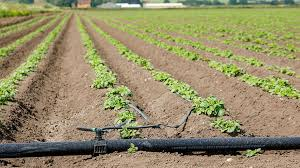The image above represents application efficiency for trickle irrigation.

To compute for application efficiency for trickle irrigation, two essential parameters are needed and these parameters are Emission Uniformity (Euand Application Efficiency for Sprinkler (Ea).

The formula for calculating application efficiency for trickle irrigation:

Eta = Eu x Ea x 100

Where:

Eta = Application Efficiency for Trickle Irrigation
Eu = Emission Uniformity
Ea = Application Efficiency for Sprinkler

Let’s solve an example;
Find the application efficiency for trickle irrigation when the emission uniformity is 32 and the applicatio efficiency for sprinkler is 4.

This implies that;

Eu = Emission Uniformity = 32
Ea = Application Efficiency for Sprinkler = 4

Eta = Eu x Ea x 100
Eta = (32) x (4) x 100
Eta = 12800

Therefore, the application efficiency for trickle irrigation is 12800%.

Calculating for the Emission Uniformity when the Application Efficiency for Trickle Irrigation and the Application Efficiency for Sprinkler is Given.

Eu = Eta / Ea x 100

Where;

Eu = Emission Uniformity
Eta = Application Efficiency for Trickle Irrigation
Ea = Application Efficiency for Sprinkler

Let’s solve an example;
Find the emission uniformity when the application efficiency for trickle irrigation is 60 and the application efficiency for sprinkler is 2.

This implies that;

Eta = Application Efficiency for Trickle Irrigation = 60
Ea = Application Efficiency for Sprinkler = 2

Eu = Eta / Ea x 100
Eu = 60 / 2 x 100
Eu = 60 / 200
Eu = 0.3

Therefore, the emission uniformity is 0.3%.

Calculating for the Application Efficiency for Sprinkler when the Application Efficiency for Trickle Irrigation and the Emission Uniformity is Given.

Ea = Eta / Eu x 100

Where;

Ea = Application Efficiency for Sprinkler
Eta = Application Efficiency for Trickle Irrigation
Eu = Emission Uniformity

Let’s solve an example;
Find the application efficiency for sprinkler when the application efficiency for trickle irrigation is 48 and the emission uniformity is 4.

This implies that;

Eta = Application Efficiency for Trickle Irrigation= 48
Eu = Emission Uniformity = 4

Ea = Eta / Eu x 100
Ea = 48 / 4 x 100
Ea = 48 / 400
Ea = 0.12

Therefore, the application efficiency for sprinkler is 0.12%.

Nickzom Calculator – The Calculator Encyclopedia is capable of calculating the application efficiency for trickle irrigation.

To get the answer and workings of the application efficiency for trickle irrigation using the Nickzom Calculator – The Calculator Encyclopedia. First, you need to obtain the app.

You can get this app via any of these means:

To get access to the professional version via web, you need to register and subscribe for NGN 2,000 per annum to have utter access to all functionalities.
You can also try the demo version via https://www.nickzom.org/calculator

Apple (Paid) – https://itunes.apple.com/us/app/nickzom-calculator/id1331162702?mt=8
Once, you have obtained the calculator encyclopedia app, proceed to the Calculator Map, then click on Agricultural under Engineering.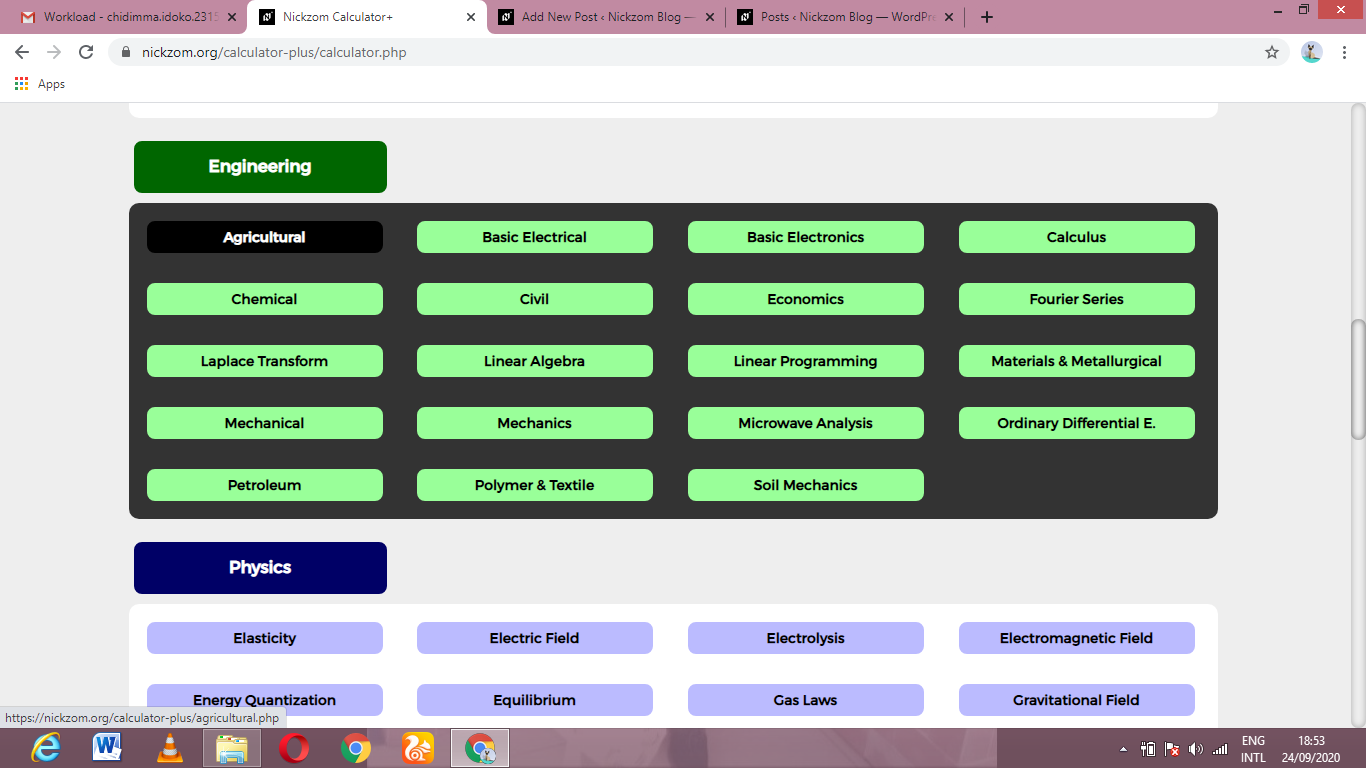Now, Click on Irrigation Water Requirement under Agricultural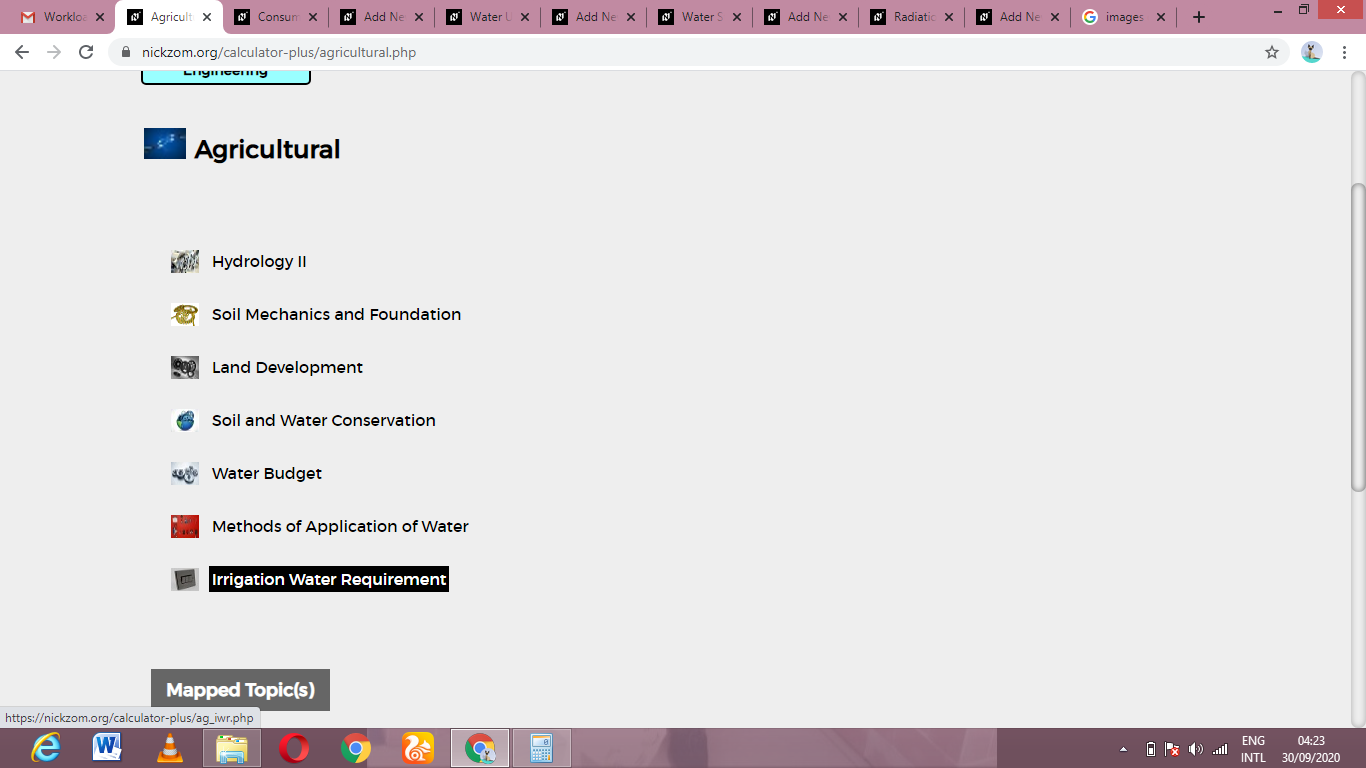Now, Click on Application Efficiency for Trickle Irrigation under Irrigation Water Requirement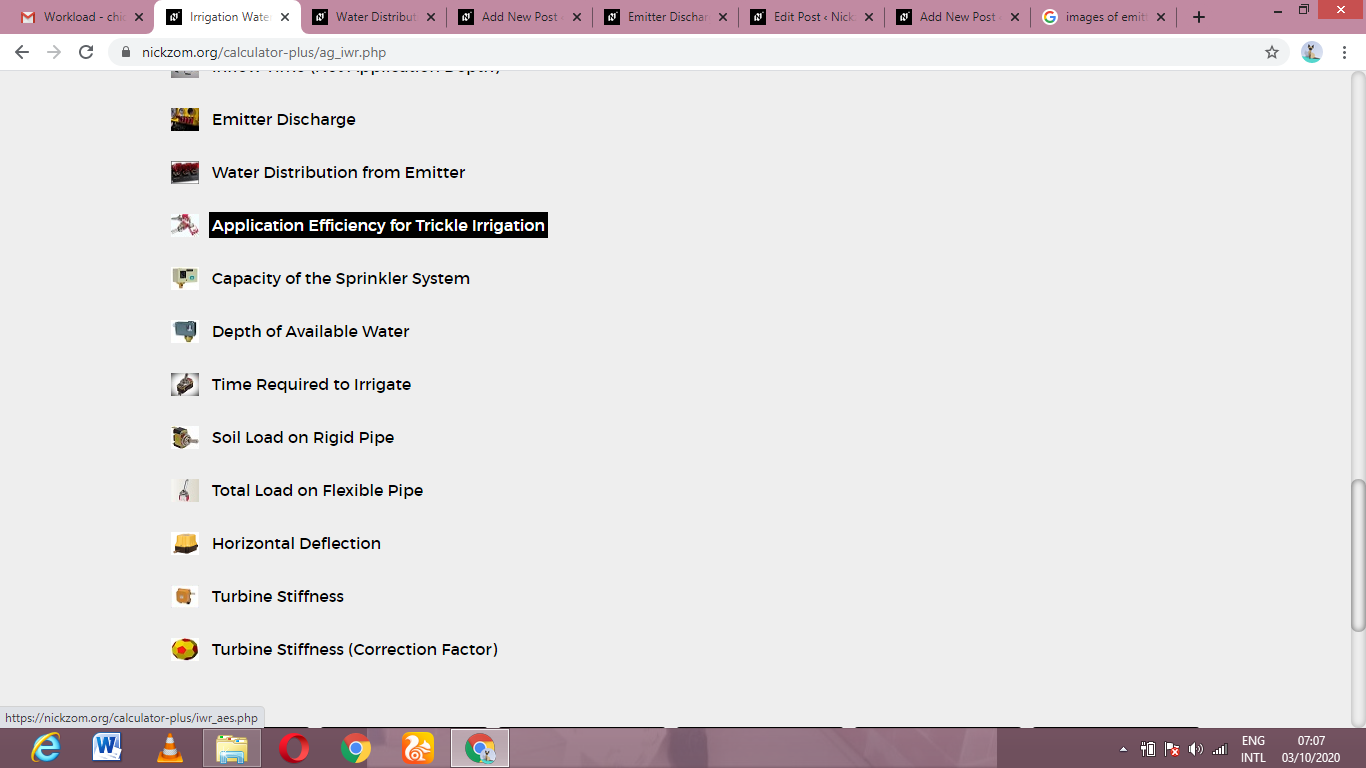The screenshot below displays the page or activity to enter your values, to get the answer for the application efficiency for trickle irrigation according to the respective parameters which is the Emission Uniformity (Euand Application Efficiency for Sprinkler (Ea).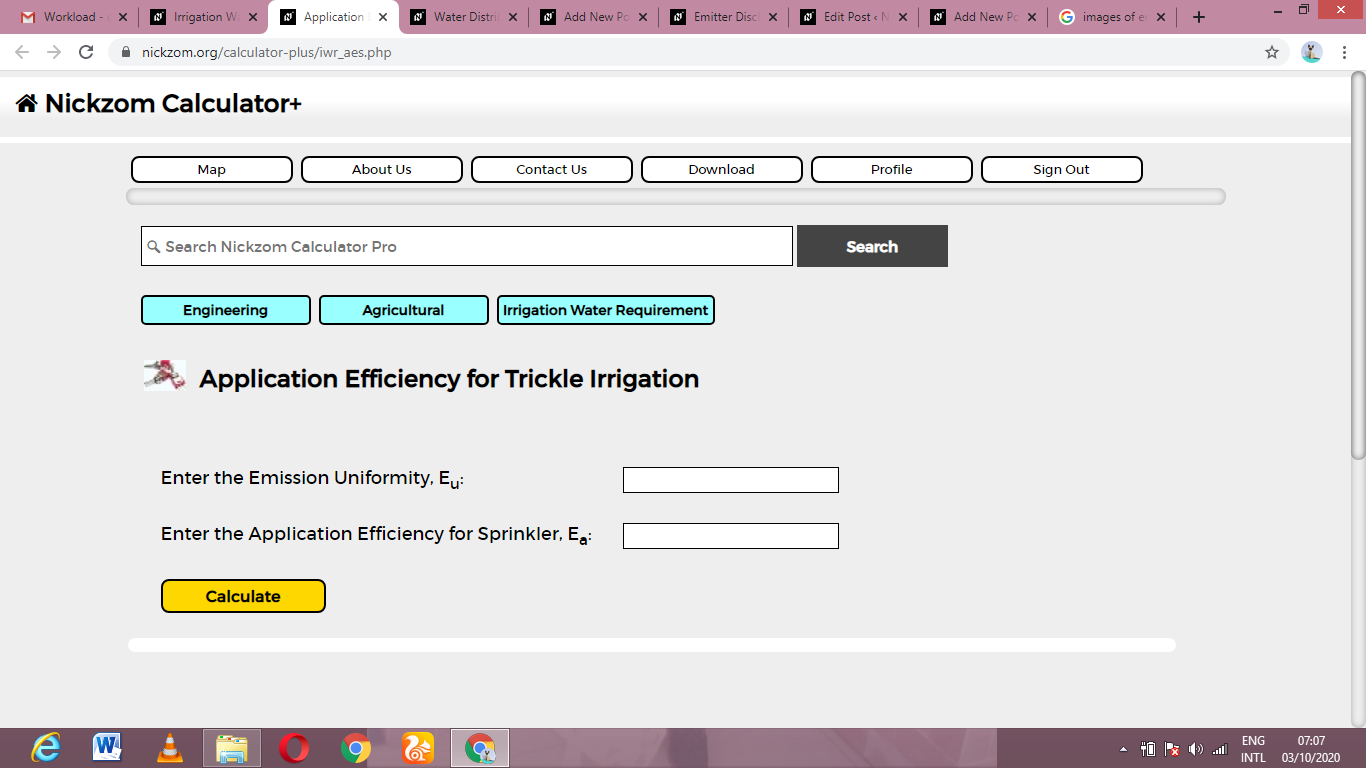Now, enter the values appropriately and accordingly for the parameters as required by the Emission Uniformity (Eu) is 32 and Application Efficiency for Sprinkler (Ea) is 4.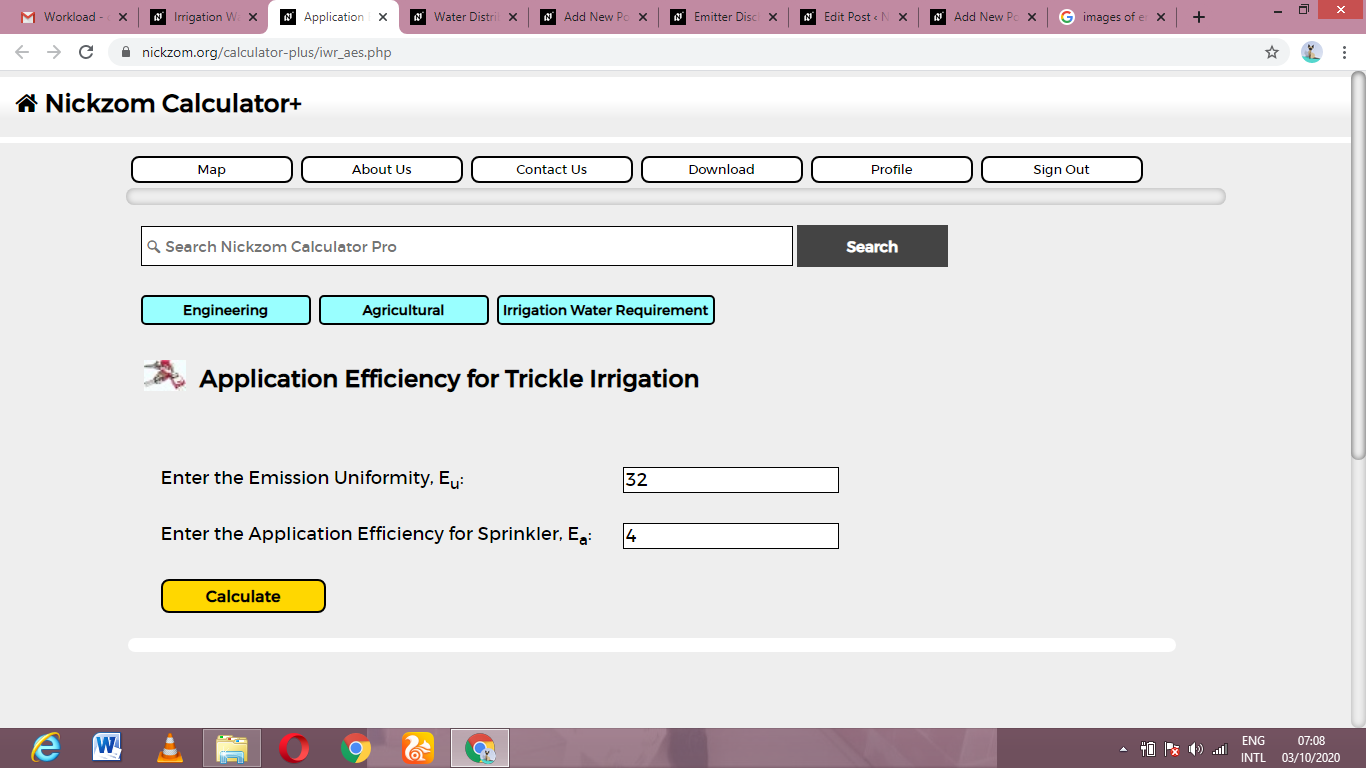Finally, Click on Calculate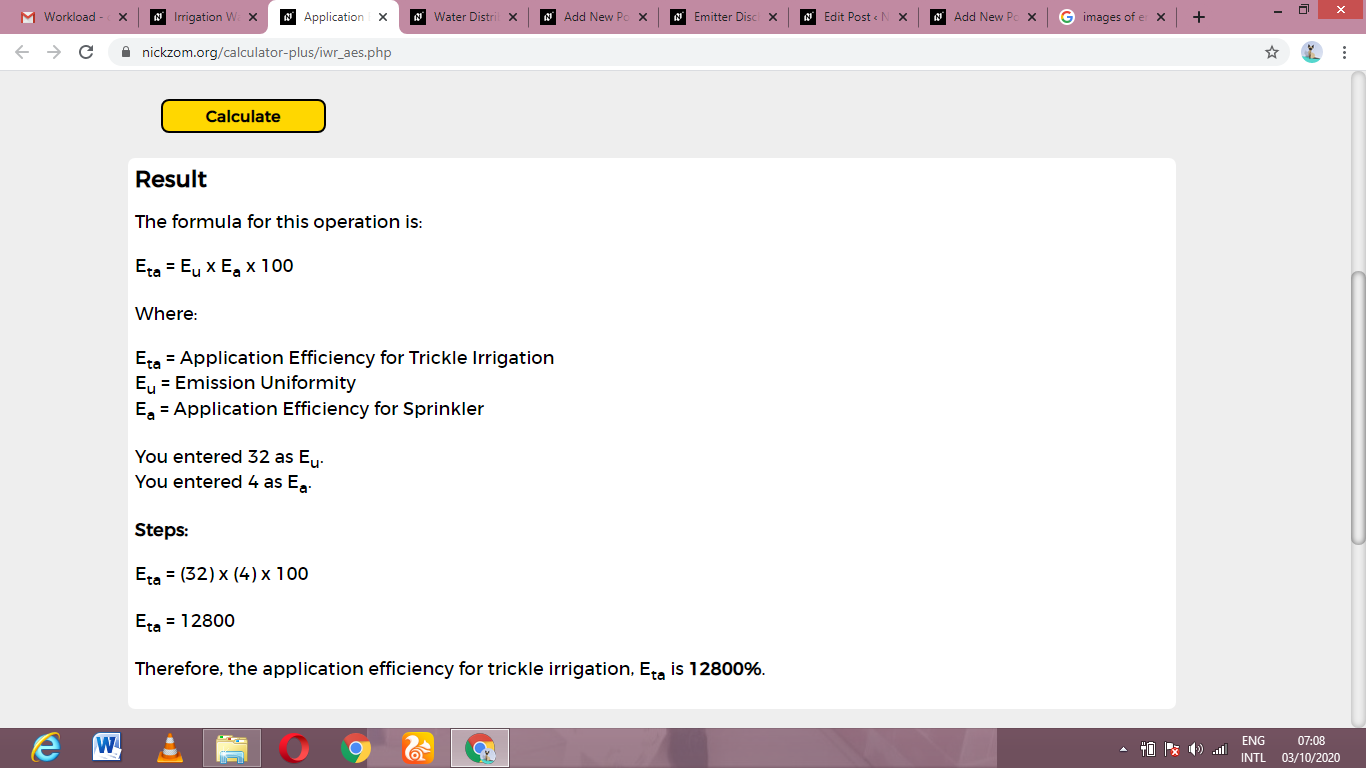As you can see from the screenshot above, Nickzom Calculator– The Calculator Encyclopedia solves for the application efficiency for trickle irrigation and presents the formula, workings and steps too.